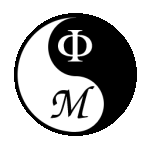Rigorous Results in Quantum Field Theory & Statistical MechanicsGENERAL
Both Quantum Field Theory (QFT) in Euclidean spacetime and Statistical Mechanics are fields of major interest in Physics. From the Mathematics viewpoint, they can be formulated as measure theories over spaces of random fields, i.e. spaces of generalized random processes.

Usually, the corresponding probability measures are built up as perturbations, about Gaussians, given by the interaction potentials either from Physics or toy models. Roughly speaking, starting from measures over (in-)finite dimensional (cutoff) spaces, Physics is concerned with the measure properties in some infinite dimension (no cutoff) limits: the ultraviolet (continuum) and infrared (thermodynamic) limits. Those limits, and so the corresponding measures, are hard to construct. The same is true if one wants to the derive the properties satysfied by the limiting measures and their moments (ergodicity properties, physical axioms, etc), i.e. correlation functions.
Led by Arthur Wightman, at the beginning, and afterwards by James Glimm, Arthur Jaffe, Ed Nelson, Tom Spencer, among others, people started attacking these mathematical problems in the 70´s. Heavy and powerful analytical methods as "cluster expansions" were developed to replace the physicist's preferred tool: usual perturbation (ordinary Taylor) expansion, almost always divergent in the above cases. These tools were pushed up in the 80's and accomodate today the renormalization group technique (a clever mixture of harmonic analysis & dynamical system methods to solve systems of coupled equations). In the last years, these methods have been succesfully exported to other fields of Mathematics.
Sure there are still plenty of open problems with the reputation of becoming more and more difficult!
To what concerns my personal contributions to the field, after a basic education in theoretical physics and a MSc degree in particle physics, I started with constructive field theory in France, at the Ecole Polytechnique. My PhD thesis was about the problem of constructing the ultraviolet limit for the tridimensional Gross-Neveu model with a large number N of fermions. This model is perturbatively nonrenormalizable but can be attacked in the framework of the 1/N expansion. It presents a nonzero fixed point solution for the renormalization group map. Afterwards, I was involved with the project of mass generation in fermionic theories. Then, we applied renormalization group techniques to analyze the critical O(N>>1) models in dimension 3. Though the original idea was to extend techniques developped in my PhD thesis to attack the full model, only some hierarchical versions were solved with success by the time being. Lately, constructive techniques have been applied to analyze the spectrum  of the time evolution operator appearing in stochastic differential equations of the Landau-Ginzburg type. Precisely, we are interested in obtaining information about two-particle bound states, what determines the approach to equilibrium of solutions to this system. Part of these projects were done in collaboration within the framework of a Mathematical Physics Pronex-CNPq grant involving colleagues from the University of São Paulo and from the Federal University of Minas Gerais. See also my OTHER ACTIVITIES PAGE.

CURRENT RESEARCH PROJECTS

My current research projects include the determination of the spectrum of dynamics generators for models in QFT, Classical Statistical Mechanics and generators associated with some Stochastic PDEs. In QFT this has to do of the determination of the particle spectrum of models. In particular, we have important results involving the Bound State Spectrum for many systems, including lattice Quantum Chromodynamics models, where the binding mechanism has been put into evidence and partial results were shown involving quark confinement.To my front page